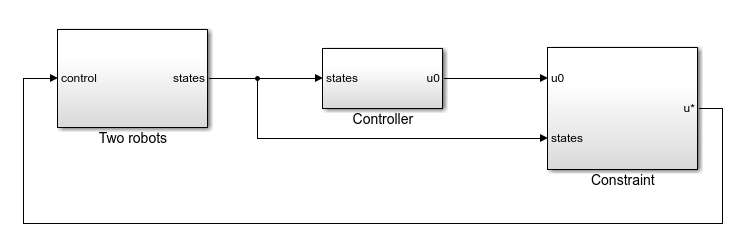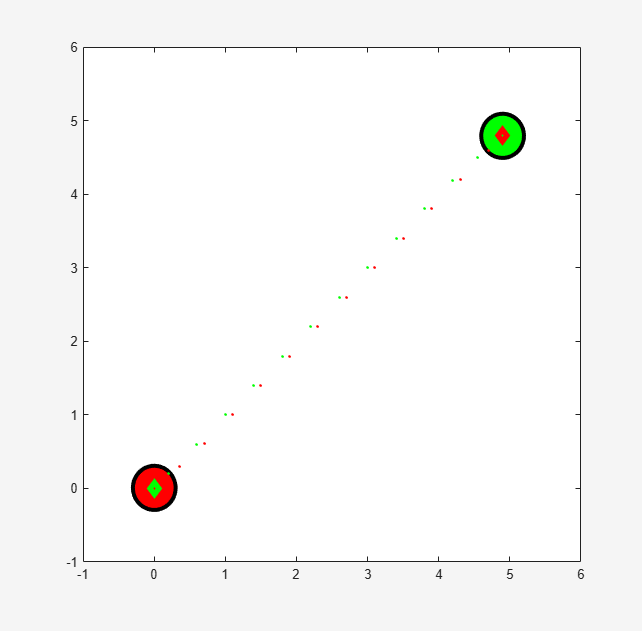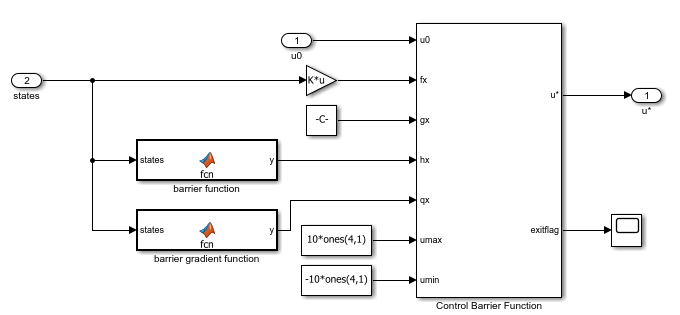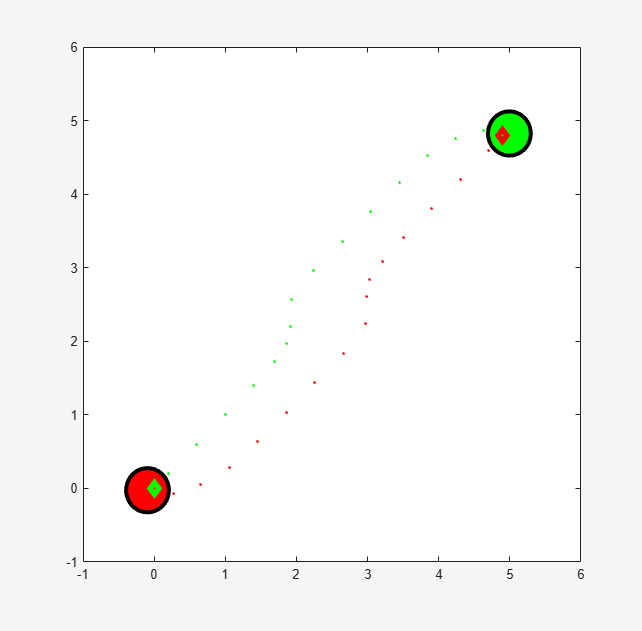# Enforce Barrier Certificate Constraints for Collision-Free Robots

This example shows how to enforce barrier certificate constraints for collision-free robots using the Barrier Certificate Enforcement block.

### Overview

In this example, the goal for the robots is to reach a target position without colliding with each other . The robot dynamics are modeled by double integrators in the x-y plane. Each robot has four states (x-position, y-position, x-velocity, and y-velocity) and two control variables (x-acceleration and y-acceleration). The velocities are constrained to [-2, 2] m/s and the accelerations are constrained to [-10, 10] m/s^2. The PID controllers are designed for the robots to reach the target position. Collision avoidance is achieved by enforcing barrier certificate constraints.

```mdl = 'twoRobotsCBF'; open_system(mdl);```### Controller Design

Each robot has its own controller that brings it to the target position. In this example, the controller is implemented as a PID-type controller.

Run the simulation and view the results.

```constrained = 0; sim(mdl);```In the figure, robot 1 (green) reaches the target position [4.9, 4.8] m and robot 2 reaches the target position [0, 0] m. The controllers successfully bring the controlled robots to their target positions.

Open the distance scope in the `Two robots` > `visualization` subsystem. The two robots collide with each other at around 1.3 seconds.### Barrier Certificate Constraints

For collision avoidance, the constraint is that the distance between two robots $\mathit{i}$ and $\mathit{j}$ stay greater than a given threshold if the two robots are moving closer to each other . The barrier certificate is given by

$h\left(x\right)=\sqrt{2{\alpha }_{sum}\left(‖▵{p}_{ij}‖-{D}_{s}\right)}+\frac{▵{p}_{ij}^{T}▵{v}_{ij}}{‖▵{p}_{ij}‖}$.

Here, the variables are:

• The maximum braking power from both robots — ${\alpha }_{\mathrm{sum}}=20$

• The minimum distance between the robots — ${\mathit{D}}_{\mathit{s}}=0.7$

• The position error vector — $▵{p}_{ij}={p}_{i}-{p}_{j}$

• The velocity error vector — $▵{v}_{ij}={v}_{i}-{v}_{j}$

The partial derivative of $\mathit{h}\left(\mathit{x}\right)$ over states $\mathit{x}={\left[{\mathit{p}}_{\mathit{i}},{\mathit{p}}_{\mathit{j}},{\mathit{v}}_{\mathit{i}},{\mathit{v}}_{\mathit{j}}\right]}^{\mathit{T}}$ is denoted by $\mathit{q}\left(\mathit{x}\right)$, and the analytical results are given in the `barrierGradFcn2Robots` script.

The Barrier Certificate Enforcement block accepts the dynamics in the form $\underset{}{\overset{˙}{x}}=f\left(x\right)+g\left(x\right)u$. For this example,

$\mathit{f}\left(\mathit{x}\right)=\left[\begin{array}{cccc}0& 0& \mathit{I}& 0\\ 0& 0& 0& \mathit{I}\\ 0& 0& 0& 0\\ 0& 0& 0& 0\end{array}\right]\mathit{x}$ and $\mathit{g}\left(\mathit{x}\right)=\left[\begin{array}{cc}0& 0\\ 0& 0\\ \mathit{I}& 0\\ 0& \mathit{I}\end{array}\right]$,

and each element is a 2-by-2 matrix.

### Simulate Collison-Free Controller with Barrier Certificate Constraint

To view the constraint implementation, open the `Constraint` > `Constrained` subsystem.

`constrained = 1;`Close the figure before running the model.

```f = findobj('Name','Two robots'); close(f)```

Run the model and view the simulation results. The two robots avoid each other when they are too close.

`sim(mdl);`The distance between the two robots stays above the threshold ${\mathit{D}}_{\mathit{s}}=0.7$.The Barrier Certificate Enforcement block successfully constrains the control actions such that the two robots reach their target positions in a collision-free manner.

```bdclose(mdl) f = findobj('Name','Two robots'); close(f)```

### References

 Wang, Li, Aaron D. Ames, and Magnus Egerstedt. “Safety Barrier Certificates for Collisions-Free Multirobot Systems.” IEEE Transactions on Robotics 33, no. 3 (June 2017): 661–74. https://doi.org/10.1109/TRO.2017.2659727.